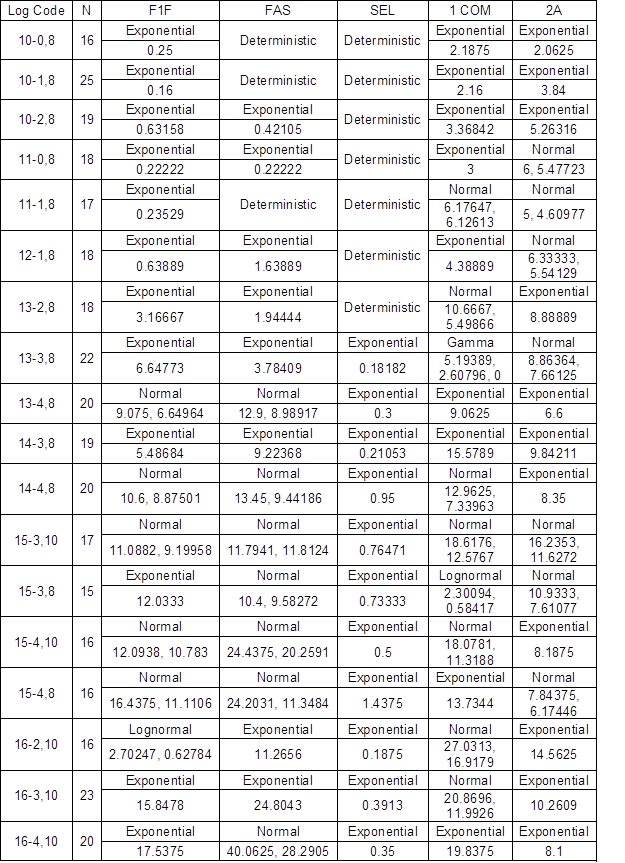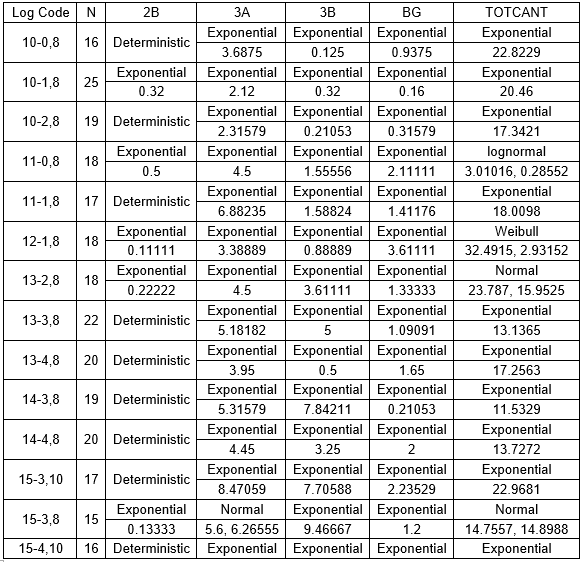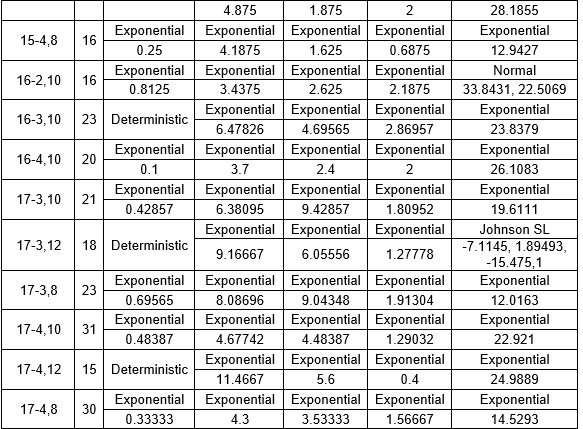Quesada, H., Adhikari, S., Bond, B., and Grushecky, S. T. (2019). "Analysis of hardwood lumber grade yields using Monte Carlo simulation," BioRes. 14(1), 2029-2050.

#### Abstract

The goal of this study was to develop a lumber grade yield prediction model with a probability-based technique known as the Monte Carlo simulation. The data to develop the prediction model was taken from an existing lumber grade yield database developed from red oak logs sawn at the Appalachian region of the United States. Statistical input analysis techniques were used to fit the lumber grade yields to hypothesized probability distributions. Inverse cumulative probability function distributions were developed from the fitted probability distributions to simulate and predict lumber grade yields. The predicted gross revenue was compared with the actual gross revenue and against the gross revenue predicted by a multiple linear regression (MLR) model. The predicted gross revenue using the Monte Carlo simulation had a 0.88% absolute error compared with the actual gross revenue, while the predicted gross revenue from the MLR model had an absolute error of 3.31%. The higher prediction power of the Monte Carlo method was more effective when predicting lumber grade yields from individual log groups. The Monte Carlo model developed in this research can be easily implemented to quickly predict lumber grade yields or gross revenue to support procurement, log inventory management, production, planning, and marketing operations.

Analysis of Hardwood Lumber Grade Yields Using Monte Carlo Simulation

The goal of this study was to develop a lumber grade yield prediction model with a probability-based technique known as the Monte Carlo simulation. The data to develop the prediction model was taken from an existing lumber grade yield database developed from red oak logs sawn at the Appalachian region of the United States. Statistical input analysis techniques were used to fit the lumber grade yields to hypothesized probability distributions. Inverse cumulative probability function distributions were developed from the fitted probability distributions to simulate and predict lumber grade yields. The predicted gross revenue was compared with the actual gross revenue and against the gross revenue predicted by a multiple linear regression (MLR) model. The predicted gross revenue using the Monte Carlo simulation had a 0.88% absolute error compared with the actual gross revenue, while the predicted gross revenue from the MLR model had an absolute error of 3.31%. The higher prediction power of the Monte Carlo method was more effective when predicting lumber grade yields from individual log groups. The Monte Carlo model developed in this research can be easily implemented to quickly predict lumber grade yields or gross revenue to support procurement, log inventory management, production, planning, and marketing operations.

Keywords: Log yield study; Monte Carlo simulation; Multiple linear regression; Probability-based model; Hardwood log yield

Contact information: a: Brooks Forest Products Center, 1650 Research Center Drive, Blacksburg, VA 24060, USA; b: Energy Land Management in the School of Natural Resources, West Virginia University,4100 Agricultural Sciences Building, P.O. Box 6108, Morgantown, WV 26506, USA;

* Corresponding author: sailesh@vt.edu

INTRODUCTION

After the United States hardwood industry production decreased from 14 billion board feet (bf) in 1999 to 6.6 billion bf in 2009, it has been showing steady signs of recovery (Snow 2017). In 2016, the United States annual hardwood lumber production reached 9.3 billion bf with the hope that the industry will continue to recover (Tucker 2017). However, it is known that United States hardwood lumber production might not be able to reach the levels that it had 20 years ago because the largest consumer of grade lumber is the furniture industry, which has moved to international locations. Additionally, the market composition for the hardwood lumber industry has changed from mainly furniture manufacturers to pallet, industrial, and export markets. Specifically, the volume of the pallet-grade lumber, industrial lumber, and export market has grown from 40% of the total market in 2008 to 77% in 2016 (Buehlmann et al. 2017; Snow 2017).

The majority of United States hardwood lumber is manufactured by small- to medium-sized sawmills that are located mostly in the southeastern region of the United States (Luppold 2015). The competitiveness of these sawmills is impacted by several external factors, including labor availability, trucking regulations, market variability, and supply chain issues. An example of supply chain issues was discussed by Cumbo et al. (2003), who studied the log quality in hardwood sawmill inventories and their overall influence on the lumber business in the southeastern region of the United States. The authors concluded that the volume of low-grade hardwood logs has increased in sawmills, consequently increasing the volume of low-grade lumber, which has potentially impacted production costs and reduced mill revenue and profit margins. Furthermore, there is little information in the literature on models that can precisely predict lumber grade yields from different log sizes and grades (Grushecky 2011), as well as the potential impact on the cost and revenue.

Previous studies on predicting hardwood lumber grade yields have been conducted using different linear regression models, such as multiple, logistic, and binary logistic models (Øvrum et al. 2009; Grushecky and Hassler 2011; Auty et al. 2014). However, prediction models that are based on regression models are limited because these models do not establish or determine fundamental relationships among the independent variables (Osborne 2015). For example, a linear regression model that uses the diameter, length, and log clear face as the independent variables to predict log yield cannot precisely predict the yield because linear regression models fail to include the interaction effect of diameter, length, and log clear face. Additionally, prediction models that are based on static inputs are not able to properly consider the random nature of the input parameters, such as the log grades, length, and diameter.

Probability-based models (PBM) are an alternative approach to regression models for developing predictions based on a known dataset. These models have a stronger prediction power because variability is considered every time the output is generated. Using a PBM to estimate log yields should provide a more accurate approach, but there is little information on the effectiveness of using PBMs to predict and analyze lumber yields from hardwood sawn logs. Therefore, the objective of this study is to apply PBM techniques, such as the Monte Carlo simulation, to simulate and analyze the lumber grade yields from hardwood sawn logs. The results of the simulated output were compared with the original output to determine the error of the PBM estimation and the prediction from a multiple linear regression (MLR) model to determine the differences. This study will help hardwood sawmills in the decision-making process by providing the information needed to optimize the benefits and allowing the opportunity to choose the quality of the logs to be processed based on the end user. The Monte Carlo model also helps to predict possible revenue from different log grades at various lumber prices with basic changes in inputs.

Hardwood lumber that is produced in sawmills is graded based on visual appearance. The grading system of hardwood lumber is overseen by the National Hardwood Lumber Association (NHLA) and is based on the board size and percentage of clear defect-free wood on the grading face(s) of the board, which must be obtained in a limited number and size of cuttings. Lumber with fewer defects is sorted as a higher grade and can be classified as First and Seconds (FAS), FAS-1-Face (F1F), and Selects, whereas lumber with a larger defect area and less clear area are sorted under 1 Common, 2A Common, 3A Common, and below. The grades, basic requirements, and potential value per board foot of the graded lumber are presented in Table 1.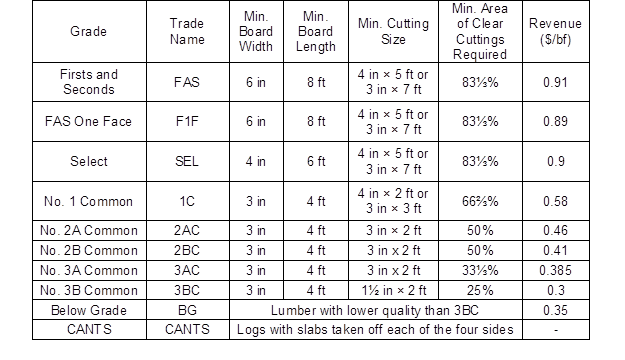Grushecky (2011)

Lumber Yield Prediction Models

Several studies using various methods to predict lumber yields have been published. Zhang et al. (2006) used regression analysis to model the product recovery in relation to selected tree characteristics for black spruce using an optimized random milling simulator. In that study, five different equations were modeled based on regression analysis, i.e., the artificial neuron network model (Agatonovic-Kustrin and Beresford 2000), lumber volume, lumber value, chip volume, and total product value. The parameters used were the diameter, height (referred to as the length in this study), and stem taper. Using the simulation, it was concluded that two of the five equation models were a good fit and the equations were able to approximately predict the actual lumber recovery, but the equations were unable to precisely predict within the expected confidence interval. Their findings revealed that the tree taper did not contribute noticeably to the product recovery and only one of the five tested regression models was preferred for estimating the product recovery. The findings from Zhang et al. (2006) also suggested that regression modeling is not appropriate for the study of lumber yields because logs have random defects in random positions. They were able to establish a mathematical relationship with the observed outputs, but the observed results failed to correctly estimate the output, which supported the fact that every correlation cannot be related to actual results (Muijs 2010).

In the study by Øvrum et al. (2009), different milling approaches were blended to develop a regression analysis model for spruce lumber output. The study used a binary logistic regression to estimate the regression coefficients and predict the probability of the outcome of lumber yields by considering the effects of the forest quality, tree size, log length, and their interactions. Øvrum et al. (2009) suggested that the log length had the strongest effect on the lumber yields when visual-strength grading rules were applied. In terms of the scaling diameter, medium-sized trees had the highest lumber yields, followed by small and then large trees. Nonetheless, the length and diameter of the logs have a strong influence on the lumber yield and revenue recovery. The findings from Rappold et al. (2007) showed that lumber yields are independent of irregular diameter geometry. The study examined lumber recovery from elliptically shaped cross-sections of red oak logs and it was found that there are no remarkable differences in the percentage of 1 COM and higher grade lumber.

Auty et al. (2014) worked on yield optimization using a model of an assortment of lumber products with zero-inflated Poisson regression. It was concluded that the number of boards produced per log depended mainly on the tree diameter and total height (length). The cited authors suggested that the final product assortment depends on the length and diameter interaction of the processed log. However, in spite of all of the other facts, the optimization approach is mainly dependent upon the quality of the log being processed. Therefore, it was concluded that a higher log quality increased the volume of high-grade lumber and process efficiencies in log processing.

In the lumber yield studies mentioned above, regression modeling was the most common approach for prediction of the lumber yields. However, regression modeling fails to explain the variability when the number of variables increases (Ott and Longnecker 2016). Additionally, with a higher in-group variability of the input variables, the prediction interval gets wider and does not support a precise estimation of the lumber yield. Another difficulty encountered when using regression is the continuously changing lumber prices (Grushecky and Hassler 2011). Because of the static input of the parameters in regression modeling, these models cannot accommodate variation in the input parameters. Therefore, parameters estimated from regression models require repeated and rigorous calculation to properly predict log yields based on changing values or revenues. The strengths and weaknesses of the various methods used to predict lumber yields are summarized in Table 2.

Table 2. Strengths and Weaknesses of the Different Lumber Yield Studies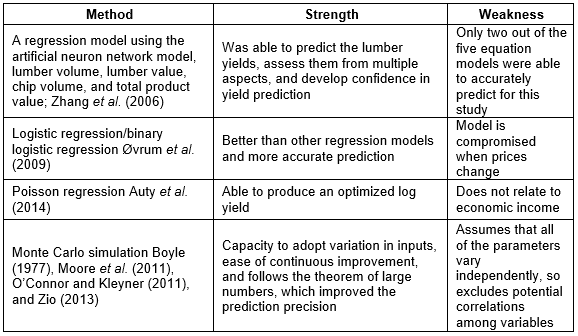Monte Carlo Simulation

Boyle (1977) indicated that the Monte Carlo simulation is a PBM that has great potential to predict log yields. The Monte Carlo simulation follows the theorem of the strong law of large numbers, which states that when there are more numbers, the predictions get closer to the population results (Nelson 2013), thus increasing its prediction precision. However, it is equally important to be aware that using the Monte Carlo simulation implicitly assumes that all of the parameters vary independently (Towler and Sinnott 2012). Therefore, it is necessary to be aware of potential correlations. According to Billinton and Li (1994), one advantage of this type of simulation is that it cannot be performed using analytical methods.

The Monte Carlo simulation has been applied to a large variety of business applications (O’Connor and Kleyner 2011), including lumber yield studies. For example, Moore et al. (2011) used this method to calculate lumber yields based on the log diameter in boreal stands in Quebec, Canada. The authors simulated a cash flow using the net present value and considered various costs from tree felling to mill processing and revenue from the lumber, wood chips, and sawdust. Then, the authors performed a sensitivity analysis with a regression of the milling method that was correlated with the net present value to identify the best scenario.

Zio (2013) indicated that the Monte Carlo simulation is one of the best tools for analyzing complex systems, such as predicting lumber yields. The simulation based on the Monte Carlo method is constructed through mathematical expressions with logical relationships that imitate the actual conditions of the studied system (Boyle 1977). In the case of a log inventory, these conditions are controlled and operated by random probabilities. The simulation is conducted using the parameters to generate values of random variables (Zio 2013). These descriptive parameters are measures of an entire population that are used as inputs in an inverse cumulative probability distribution function (ICPDF).

Statistical input analysis or data fitting to a probability distribution function (PDF) is the preliminary step before obtaining an ICPDF. Observed responses, such as lumber yields, are tested against expected values from a hypothesized probability distribution. The overall test of the fitted distribution is based on the level of adjustment that exists between the frequency of the observations in a given sample and the expected frequencies that are obtained from the hypothetical distribution. A significance level is set to test the null hypothesis that the data comes from the hypothesized distribution. If the null hypothesis is rejected, it is indicated that the data does not come from the hypothesized distribution (Hogg et al. 2015; JMP 2018).

According to Boyle (1977), the main disadvantage of PBM models, such as the Monte Carlo model, is that these methods assume that all of the input parameters are independent and correlations are not present. However, in the previous lumber yield studies discussed above, it was found that the input parameters, such as the length, diameter, and log grades, are not independent and they all have to be considered when predicting log yields.

Thus, the objective of this paper is to conduct Monte Carlo simulation and develop an MLR equation based on existing log yield data to analyze log yield output based on visual grading of hardwood logs and compare the results with an actual yield to measure the effectiveness of the purposed method.

EXPERIMENTAL

Methodology

The list of steps and methods performed in this study are shown in Fig. 1. The lumber grade yield data used for this research was developed by Grushecky and Hassler (2011). This database includes lumber grade yields for 1472 red oak logs that were divided into 214 log groups (defined by clear face grade, length, and scaling diameter). The grade referred to the number of clear faces (0 to 4), the length was given in feet, and the diameter (small end) was in inches using the Doyle and International scale rules. The lumber output was recorded in board feet under the following NHLA classifications: FAS, F1F, 1 COM, 2A COM, 2B COM, 3A COM, 3B COM, BG, SEL, and CANT. These 10 lumber grades were used as the subgroups of the log yields in this dataset.

The logs from the dataset were rearranged and classified into groups by grade, length, and scaling diameter. For example, a log group with the notation 18-2,12 would have an 18-in small end diameter, two clear faces, and a 12-ft length. The second step was to remove all of the log groups with a sample size smaller than 15 from the dataset. It was decided that smaller sample sizes were not appropriate for obtaining valid results. These two steps were conducted using Microsoft Excel (2016 Microsoft Office, v. 16.0, Redmond, WA, USA).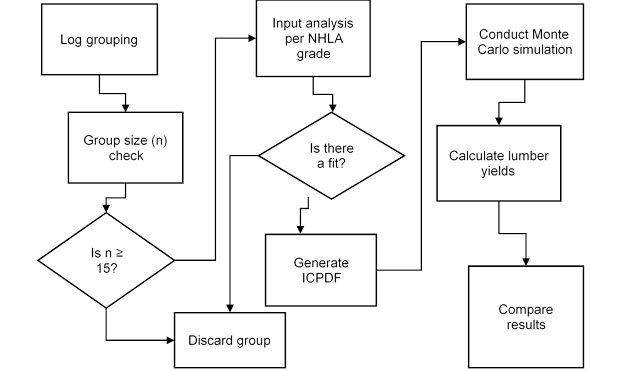Fig. 1. Flowchart of the methodology

The third step required using statistical input analysis to fit the lumber grade yields (board feet by NHLA grade for each group) to a hypothesized probability distribution. The SAS/JMP software (SAS-JMP® Pro, version 13.0.0, Cary, NC, USA) was used for this step. A goodness of fit test with a 5% significance level was used to select the best possible fit. In the cases where a lumber grade (within a log group) had a sample size of less than three, it was not possible to fit a probability distribution; therefore, the data were considered deterministic and the observed mean was used as the parameter.

The best probability distribution was fitted to obtain a probability distribution function (PDF) of each lumber grade yield for each log group. The PDF obtained from the distribution with its parameter was used to develop ICPDF in Excel. The implementation of the ICPDF in Excel required the parameters of the fitted probability distribution and a random number between ‘0’ and ‘1’. For example, for a fitted normal ICPDF, the parameters are the mean and standard deviation, while for a fitted exponential ICPDF, only the mean is required. The ICPDF for normal distribution with mean ‘m’ and standard deviation ‘σ’ was evaluated as NORM.INV (RAND (), m, σ). For some ICPDFs, Excel already includes a built-in formula, but for the others, ICPDF formulas were developed and implemented by the authors when necessary.

The next step was to conduct the Monte Carlo simulation using the fitted ICPDFs and their parameters. For each NHLA grade of each log group, 1000 replicas were generated, as was suggested by the law of large numbers (O’Connor and Kleyner 2011).

The simulated lumber grade yields were multiplied by their reference price to determine the total gross revenue per log group. Lumber prices were taken from the industry reports used in the study by Grushecky and Hassler (2011). The simulated lumber grade yields and gross revenue values were compared with the actual values in the original lumber grade yields database. Additionally, the lumber grade yields and gross revenue were estimated through an MLR for comparison purposes. The MLR model was developed using the length, scaling diameter, and log grade as the input variables, as was indicated in previous lumber yield studies.

RESULTS AND DISCUSSION

Average Log Yields by Log Group and Lumber Grades

Only log groups in the log grade yield dataset with a sample size equal to or greater than 15 were considered for further analysis. This data showed that when the log (diameter and length) was larger and the grade was higher, more revenue was obtained as more volume and higher-grade lumber was produced.

Model Fitting for the Monte Carlo Method

The first step in estimating the prediction model for the Monte Carlo method required an input analysis (a procedure to fit the data to a hypothesized probability distribution) for the log groups in the study. Therefore, 240 lumber grade yields were analyzed using the statistical software SAS/JMP to fit a PDF. A summary of the fitted PDFs is presented in Fig. 2. The majority of fitted PDFs was exponential with 172 cases (71.7%), and the second most fitted distribution was the normal PDF with 33 cases (13.7%). These two PDFs represented 85.4% of all of the cases. There were 24 cases where a PDF was not fitted because there was not a large enough sample size (greater than and equal to 3) and therefore, a deterministic fit was used. The specific fitted PDFs with their respective parameters, by lumber grade and lumber group, are included in Table A1.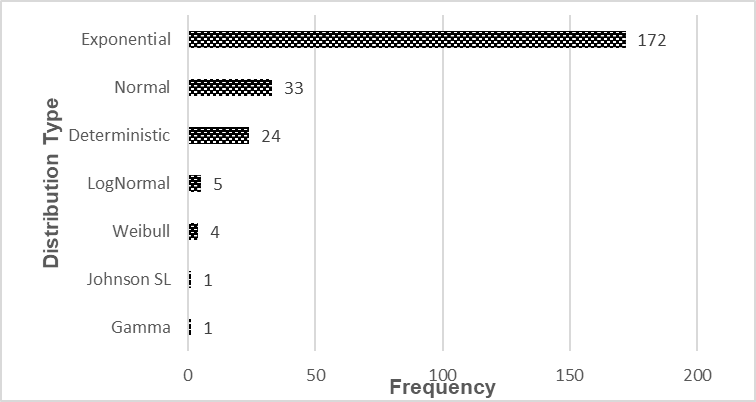Fig. 2. Frequency of the probability distributions of the log yields

Once the data were fitted to a PDF, the next step required obtaining an ICPDF for each of the fitted PDFs. The ICPDF and parameters determined for each were used to simulate the log yields following the Monte Carlo method. A total of 1000 iterations were simulated for each lumber grade of each log group. The averages of the predicted values using the Monte Carlo method are shown in Table 4. The column “\$/log” shows the average revenue per log group. The revenue per log was obtained by multiplying the amount of board footage of each grade by its corresponding market value.

Table 3. Average Log Yields by Grade for Each Log Group in the Analysis and Revenue Per Log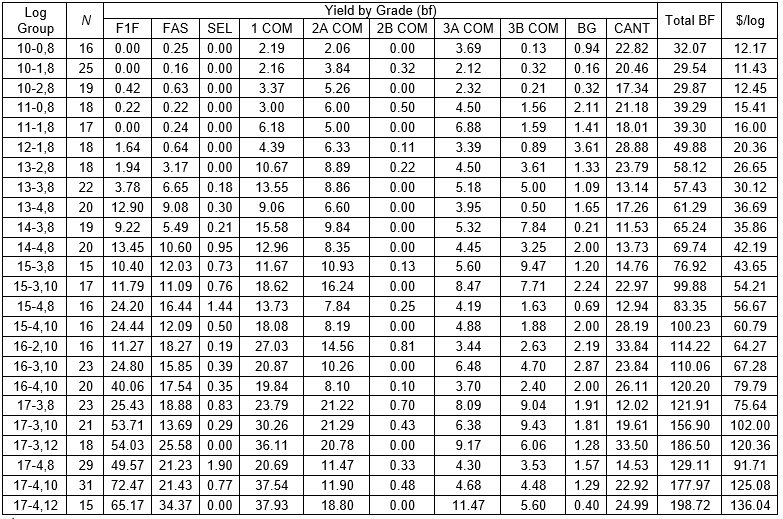– sample size

Table 4. Log Yields by Grade and Log Group from the Monte Carlo Simulation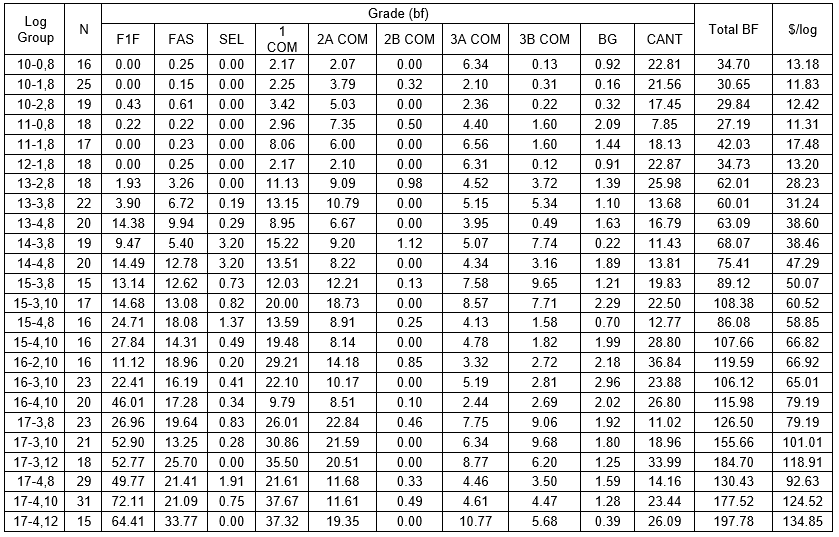Model Fitting Using Linear Regression

In addition to developing a model to predict the log yields and revenue using the Monte Carlo method, an MLR model was implemented with the goal of comparing both methods with the real lumber yields. According to the results in the literature, the length, diameter, and log grade can be used as predictors for the log yields. Therefore, the data from the same 24 log groups used to simulate the log yields with the Monte Carlo method were used to estimate the coefficients of the MLR models. The coefficients to predict the board footage by grade are shown in Table 5 and statistical output is presented in Appendix in Table A1 to Table A3.

Table 5. MLR Coefficients by Grade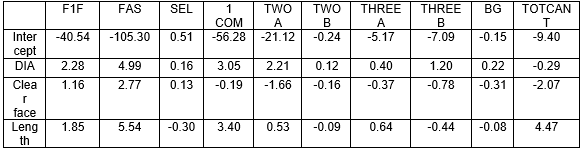For each log group (diameter, clear face, and length), the corresponding intercept and coefficients were used to estimate the board footage by grade. The revenue per log was calculated using the grade market prices, as in previous calculations. The predicted board footage for each log group and revenue is displayed in Table 6. This MLR model had a decent fit as the R2value observed was 0.687 with p-value less than 0.0001. This low P-value and moderate R2 value indicates that the deviations in the predictors were correlated to alterations in the response variable. Thus this MLR model explained substantial response variability based on variation in input parameter. Also, the adjusted R2, the proportion of total variance that is explained by MLR model, was 0.685. With three predictors and adjusted R2 value of 0.685 indicates a good fit of the model. Additionally, the root mean square error of the whole model was 23.92, which is considerably lower spread of prediction error, supporting the good fit of the MLR model.

To estimate the yield with the MLR model, multicollinearity between variables can be the major limiting factor for precise outputs. To estimate the precise yield from MLR equation, all the variables in a regression model should behave independently. To understand the effect of correlation among variables, the Variance Inflation Factor (VIF) of the MLR model was observed. Diameter has the highest VIF of 3.3, the clear face has VIF value of 2.4 and length has lowest VIF of 1.63. This observation indicated that all three variables were moderately correlated as per the rule of the thumb described by Ott and Longnecker (2016). The results can be interpreted as the prediction including the diameter of the logs will predict 230 % bigger value compare to the predicted value if there was no correlation between diameter, clear face, and length of the logs. As VIF for this prediction model is considerably lower than 5, multicollinearity will not be the major limitation to predict the lumber yield. As the VIF of the variables is moderately correlated, it also fulfills the assumption of Monte Carlo simulation as independent variables.

Table 6. Log Yields by Log Group and Grade from MLR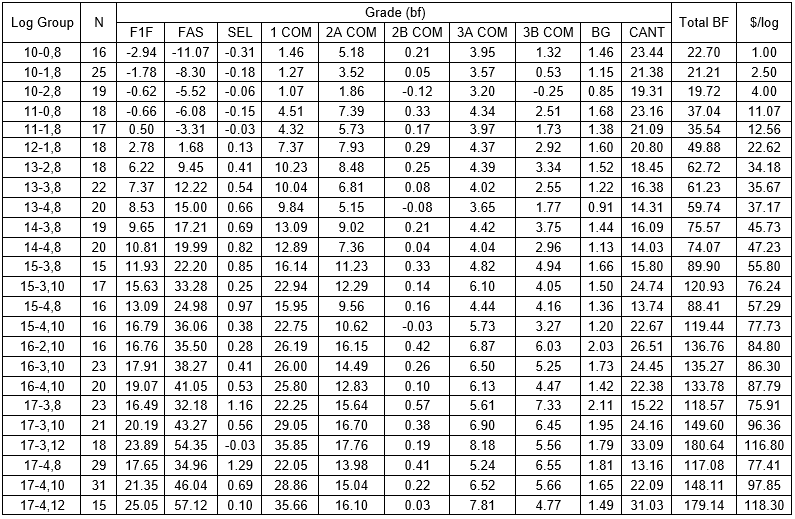Comparisons of the Predicted Values with the Real Log Yields

The average gross revenue per log group from the MLR, Monte Carlo method, and real values are shown in Table 7. The maximum error produced when using the Monte Carlo method was found for log group 12-1,8 at 35% and the second largest error was for log group 11-0,8 at 27%. Out of the 24 log groups used in the analysis, 16 log groups had an error of less than 10% when using the Monte Carlo method. The prediction for log groups 16-2,10 to 17-4,12 had a maximum error of 6% (log group 16-3,10) and a minimum error of 0% (log group 17-3,12). This suggested that when the log quality was higher and the diameter and length were larger, a more accurate prediction of the log yield was obtained using the Monte Carlo method.

Table 7. Comparison of the Predicted Log Grade Yields in Gross Revenue with the Real Values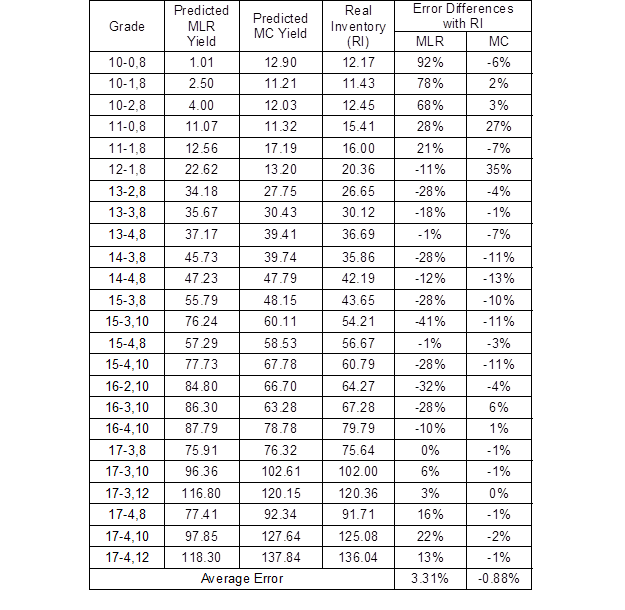MC – Monte Carlo

The prediction results from the MLR by log group were less precise than the results obtained from the Monte Carlo method (Table 7). The maximum prediction error when using the MLR method was found for log group 10-0,8 with an absolute error of 92%. The second largest error was found for log group 10-1,8 at 78%, and the third largest error was for log group 10-2,8, which was 68%. This suggested that the MLR had less accuracy than the Monte Carlo method when the log quality and dimensions were smaller. However, when the quality and dimensions of the log groups were higher, the MLR had a higher prediction accuracy range of 22% to 13% for the top three log groups (in terms of the quality and dimensions). Additionally, the MLR prediction of the log grade yields by gross revenue had only five log groups with absolute errors of less than 10%.

In summary, when both prediction models were compared against the total average real log yield revenue, it was found that the MLR model had an overall error of 3.31% and the Monte Carlo method had an error of -0.88% (Table 7). This indicated that as an aggregate, both models performed well, but the resulting data suggested that it was better to look at each individual log group rather than the aggregate data. Finally, Fig. 3 shows the revenue trend for both models and the real lumber yields as the grade and size of the logs increased.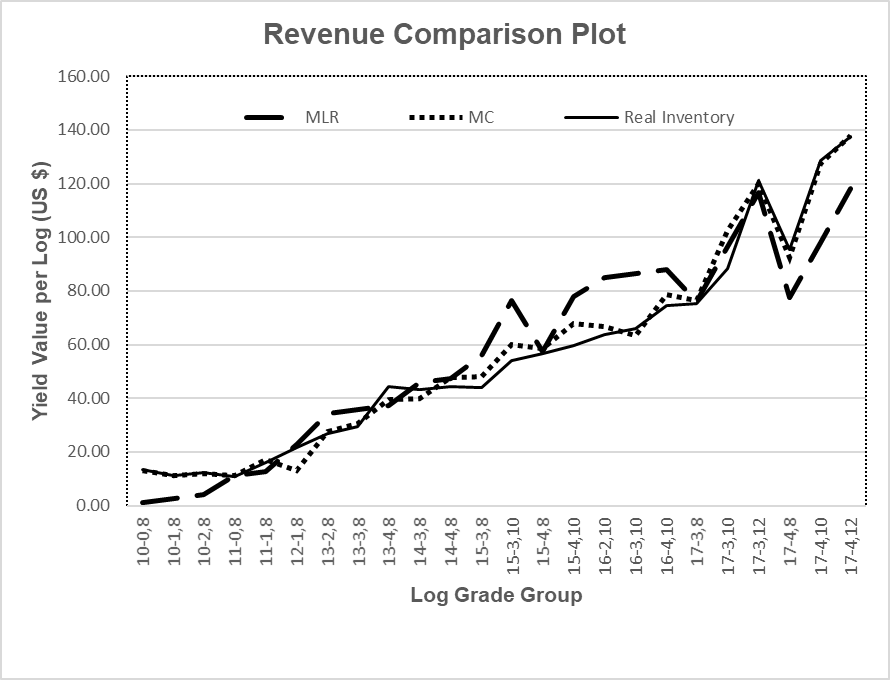Fig. 3. Revenue comparison plot based on the log yield prediction from the Monte Carlo method and MLR vs. the real inventory

This research provides an important step to assist hardwood sawmills in developing better models to estimate lumber yields based on specific log characteristics. Log grading systems are designed with the goal of separating or classifying logs by the potential gross revenue based on the lumber grade yields. A common agreement among lumber producers and academics is that lumber grade yields are highly variable, but there are some fundamental characteristics, such as the scaling diameter, length, and log grade, that highly correlate with lumber yields. However, the difficult task of predicting log grade yields from the intrinsic yield variability is still one of the main drivers for the industry to estimate the gross revenue or profitability over an extended period of time and large log batches, rather than focusing on the potential gross revenue from individual logs or smaller log batches.

Historically, hardwood sawmills have not been enthusiastic about adopting new information from previous lumber yield studies due to a lack of resources and the complexity. Nevertheless, with the advancement of information technologies, data analytics have taken a step forward to develop quicker, more effective, and simple data prediction models that can support sawmills in making better decisions to quickly react to changes in pricing or a lack of raw material. In this study, a model was developed to estimate lumber grade yields based on the Monte Carlo simulation. This method does not force the data to a specific deterministic equation, but rather fits the data to a probability distribution that can explain variability better than deterministic models. By hypothesizing that the behavior of each lumber grade yield is different, the implementation of the Monte Carlo method revealed that lumber grade yields behave differently for each lumber grade. In this study, input analysis was applied to a set of 24 log groups each with 10 different lumber grades, which resulted in a variety of fitted PDFs. The need to use a variety of distribution functions showed that lumber grade yields are associated with many different variables that are difficult to separate and analyze on their own.

In contrast to the Monte Carlo method, the MLR model predicted lumber grade yields and gross revenue as a single point estimator. For lumber grade yields, the MLR model had a better prediction accuracy than other linear regression models, but the major problem with this type of estimation was the limitation of the model in incorporating variability. It was found in this research that estimated MLR equations were unable to explain the basic relationship of the variables and outcomes. For example, log group 10-0,8 had a predicted error of 92%, but the prediction errors for log groups 13-4,8 and 15-4,8 were only 1% of the real gross revenue. These results suggested that the MLR increased its prediction accuracy only when the value of the variables (diameter, clear face, and length) were closer to their mean values.

The results also indicated that an estimation of the lumber grade yields based on the grade depended on the dimensions and quality of the log. Differences in the gross revenue of different log qualities and dimensions were evident. This finding was similar to that of other research, which has concluded that the most important factors of the yield recovery are the diameter, length, log grade, milling methods, and forest site conditions. Though not all of these variables were used in this research, the findings still reinforced what has been reported in previous literature. Additionally, this study only included log groups with sample sizes equal to or greater than 15. Thus, it is necessary to include additional log groups with higher sample sizes in future research if possible.

Finally, the results of this study could be used by practitioners to estimate with high confidence the potential revenue from a specific set of logs or log inventory that matches the log groups analyzed in this study. An analytical tool can be easily developed in Excel that can perform the simulation for a particular log inventory.

CONCLUSIONS

1. The input from the statistical analysis applied to lumber grade yields from 24 log groups with sample sizes equal to or greater than 15 indicated that out of the 240 lumber grade yields used in the analysis, 172 fit an exponential distribution (71.7%) and 33 fit a normal distribution (13.7%). These two distributions represented 85.4% of all of the cases.
2. When the Monte Carlo method was applied to predict the gross revenue by the log groups, the results indicated that 16 of the 24 log groups had a gross revenue error of less than 10%. In contrast, the MLR model results indicated that only five groups out of the 24 log groups had an error of less than 10%. This implied that the accuracy of the Monte Carlo method to predict the gross revenue from individual log groups was higher than that of the MLR method.
3. The highest error when predicting the gross revenue using the Monte Carlo method was found for log group 12-1,8 at 35% for the real gross revenue. Meanwhile, the highest error when using the MLR method was found for log group 10-0,8 with a 92% error when compared with the real gross revenue.
4. When the errors for all of the log groups in the analysis were combined, the Monte Carlo method had a 0.88% error (absolute) and the MLR method had a 3.31% error (absolute). This indicated that when log groups were analyzed together, both methods had a good estimation ability.
5. A simulation model for the log yield can be adjusted to price changes and self-adapt the probable variability to evaluate the possible revenue recovery in the least confidence interval.
6. The dynamic nature of the Monte Carlo simulation makes it superior to regression models that have been used in the past to predict lumber grade yields.

ACKNOWLEDGMENTS

The authors are very grateful to Dr. Curt Hassler and the Appalachian Hardwood Center at West Virginia University for the temporary loan of their proprietary log and lumber yield data, allowing us to conduct this research. This work was funded through the grant USDA-Forest Service 16-DG-11083150-053.

REFERENCES CITED

Agatonovic-Kustrin, S., and Beresford, R. (2000). “Basic concepts of the artificial neural network (ANN) modeling and its application in pharmaceutical research,” J. Pharmaceut. Biomed. 22(5), 717-727. DOI: 10.1016/S0731-7085(99)00272-1

Auty, D., Achim, A., Bédard, P., and Pothier, D. (2014). “StatSAW: Modelling lumber product assortment using zero-inflated Poisson regression,” Can. J. Forest Res. 44(6), 638-647. DOI: 10.1139/cjfr-2013-0500

Billinton, R., and Li, W. (1994). “Elements of Monte Carlo methods,” in Reliability Assessment of Electric Power Systems using Monte Carlo Methods, Springer, New York, NY, pp. 33-73.

Boyle, P. P. (1977). “Option: A Monte Carlo approach,” J. Financ. Econ. 4, 323-338.

Buehlmann, U., Bumgardner, M., and Alderman, D. (2017). “Recent developments in United States hardwood lumber markets and linkages to housing construction,” Current Forestry Reports 3(3), 213-222. DOI: 10.1007/s40725-017-0059-y

Clark, S. L., Schalarbaum, S. E., and Kormanik, P. P. (2000). “Visual grading and quality of 1-0 northern red oak seedlings,” South. J. Appl. For. 24(2), 93-97. DOI: 10.1093/sjaf/24.2.93

Cumbo, D., Smith, R., and Araman, P. (2003). “Low-grade hardwood lumber production, markets, and issues,” Forest Prod. J. 53(9), 17-24.

Denig, J., Wengert, E. M., Brisbin, R., and Schroeder, J. (1984). “Dimension lumber grade and yield estimates for yellow-poplar,” South. J. Appl. For. 8(3), 123-126. DOI: 10.1093/sjaf/8.3.123.

Grushecky, S. T. and Hassler, C. C. (2011). “A non-hierarchical clustering and ordinal logistic regression approach to developing red oak log grades in the Central Appalachian region,” in International Scientific Conference on Hardwood Processing, Blacksburg, VA, USA.

Hogg, R. V., McKean, J. W., and Craig, A. T. (2015). “Optimal tests of hypotheses,” in An Introduction to Mathematical Statistics, Pearson Education, Inc., London, UK, pp. 429-472.

JMP- statistical software- (2018). “Fitting distributions,” JMP Statistical Discovery, (https://www.jmp.com/content/dam/jmp/documents/en/academic/learning-library/03-fitting-distributions.-pdf), Accessed 20 Aug 2017.

Kretschmann, D. E. (2010). “Mechanical properties of wood,” in Wood Handbook – Wood as an Engineering Material (FPL-GTR-190), U.S. Department of Agriculture Forest Products Laboratory, Madison, WI.

Luppold, W. (2015). “The North American hardwood market: Past, present, and future,” in 2nd International Scientific Conference on Hardwood Processing, Paris, France.

Mayer, R., and Wiedenbeck, J. (2005). Continuous Sawmill Studies: Protocols, Practices, and Profits (General Technical Report NE-334), U.S. Department of Agriculture Northeastern Research Station, Newton Square, PA.

Moore, T. Y., Ruel, J.-C., Lapointe, M.-A., and Lussier, J.-M. (2011). “Evaluating the profitability of selection cuts in irregular boreal forests: An approach based on Monte Carlo simulations,” Forestry 85(1), 63-77. DOI: 10.1093/forestry/cpr057

Muijs, D. (2010). Doing Quantitative Research in Education with SPSS (Second Edition), Sage Publications, London, UK.

Nelson, B. L. (2013). Foundations and Methods of Stochastic Simulation: A First Course, Springer, New York, NY.

O’Connor, P. D., and Kleyner, A. (2011). Practical Reliability Engineering (Fifth Edition), John Wiley & Sons, Hoboken, NJ, USA.

Osborne, J. W. (2015). Best Practices in Logistic Regression, Sage Publications, London, UK.

Øvrum, A., Høibø, O. A., and Vestøl, G. I. (2009). “Grade yield of lumber in Norway spruce (Picea abies (L.) Karst.) as affected by forest quality, tree size, and log length,” Forest Prod. J.59(6), 70-78.

Ott, R. L., and Longnecker, M. T. (2016). An Introduction to Statistical Methods and Data Analysis (Seventh Ed.), Cengage, Boston, MA, USA.

Rappold, P. M., Bond, B. H., Wiedenbeck, J. K., and Ese-Etame, R. (2007). “Impact of elliptical shaped red oak logs on lumber grade and volume recovery,” Forest Prod. J. 57(6), 70-73.

Snow, M. (2017). “American hardwood export,” American Hardwood Export Council, (https://www.lumberclub.org/wp-content/uploads/SnowCharlotte0917-web.pdf), Accessed 10 May 2018.

Taylor, A. (2007). A Hardwood Log Grading Handbook, University of Tennessee, Knoxville, TN, USA.

Towler, G., and Sinnott, R. K. (2012). “Process simulation,” in Chemical Engineering Design: Principles, Practice, and Economics of Plant and Process Design, Butterworth-Heinemann, Oxford, UK, pp. 161-207.

Tucker, R. (2017). “Hardwood: State of the industry—Housing sector, engineered sales drive revenues,” Floor Covering News, (http://www.fcnews.net/2017/03/hardwood-state-of-the-industry-housing-sector-engineered-sales-drive-revenues/), Accessed 28 Aug 2017

Zhang, S. Y., Liu, C., and Jiang, Z. H. (2006). “Modeling product recovery in relation to selected tree characteristics in black spruce using an optimized random sawing simulator,” Forest Prod. J. 56(11-12), 93-99.

Zio, E. (2013). The Monte Carlo Simulation Method for System Reliability and Risk Analysis, Springer-Verlag, Dordrecht, Germany.

Article submitted: August 16, 2018; Peer review completed: December 15, 2018; Revised version received: December 27, 2018; Accepted: December 28, 2018; Published: January 25, 2019.

DOI: 10.15376/biores.14.1.2029-2050

APPENDIX

Table A1: Log Types and Correspondent Probability Distribution for Each Grade